# Factors Affecting Friction

• Last Updated : 21 Feb, 2021

Friction is an opposing force that restricts the motion of one body over the surface of another body in contact with it. It is absolutely necessary for the motion of any object over another. It is like a necessary evil which harms and helps. Its dual function can be understood by the example of walking, we are able to walk because there is friction, and we are walking at a certain low speed because of the opposing force provided by friction.

Also, Like in case of a ball freely rolling or sliding on the ground, the ball eventually comes to stop, because of the force of friction that acts between the ball and the ground during its movement. This means that if a force acts in the direction from right to left, friction acts in left to right direction and the vice versa is also true that if the force acts in left to right the friction will act in the opposite direction that is from right to left.

Attention reader! All those who say programming isn't for kids, just haven't met the right mentors yet. Join the  Demo Class for First Step to Coding Course, specifically designed for students of class 8 to 12.

The students will get to learn more about the world of programming in these free classes which will definitely help them in making a wise career choice in the future.

There are some forces which only comes into action when they are in contact with each other. It is known as the contact forces. It is offered by solids, liquids and also by gases, but it is maximum in the case of solids and least in the case of gases. The effects of friction include the gradual wearing away of things; this can be seen in examples like the uneven soles of our shoes, balding bicycle tyres and the deliberate action of rubbing sandpaper on a surface.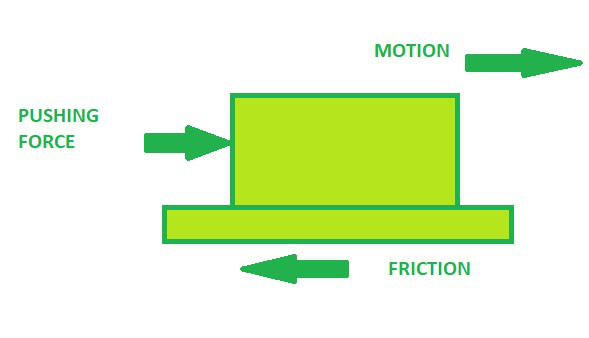Friction can only be reduced it cannot be eliminated. We can reduce friction with the help of lubricants on the surface in contact. Reducing friction helps in better movement of objects over each other. If a surface is very rough we need to make it smoother for movement using some effective lubricants such as oil or grease like incase of machinery parts we use greasing method to avoid friction between the parts when functioning.

If we reduce friction to zero, movements will not take place as then the object will not move it will just slip over the surface of the other and hence it is required to have some minimum friction for any kind of motion to take place between two objects or surfaces. Like for example, we cannot walk in ice because its surface is smoother and so it has the least friction and hence we slip whenever we try to walk there. Hence, for ice skating or any other activity on the surface of ice we need to use specially designed ice skating shoes or boots which have ends with rough surfaces to ensure we have some required friction for our movement.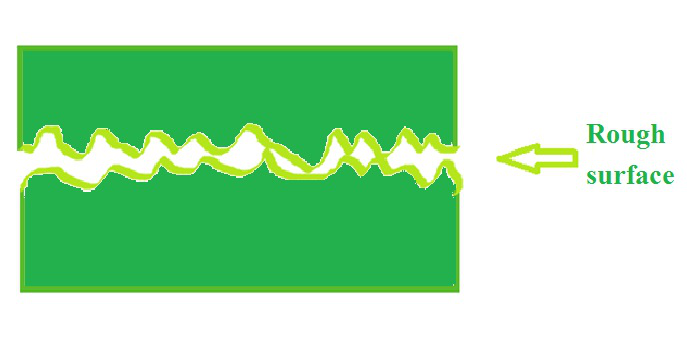Surfaces in Contact

### What causes Friction?

Friction is a force that resists or opposes the relative motion between two objects or materials. Various reasons are responsible for this opposing force to come into action. Among various other causes, the main cause of this resistive force or frictional force is molecular adhesion, surface roughness which depends on the nature of surface and body in contact, and deformations in the surface or in the moving object.

1. Adhesion is the molecular force resulting when two materials are brought into close contact with each other. Like there is a force of adhesion between the surface of glass and water that is why when poured into a glass it wets the surface of the glass.
2. Every object has a rough surface irrespective of it looking smoother in our naked eyes. But when we observe through a microscope, we find that the surfaces of all the objects have rough edges. And hence two rough surfaces, when come in contact with each other, generate frictional force or an opposing force which sometimes may convert into heat between them. The degree of friction increases when the roughness increases as it is harder to walk on a road with many pebbles and easier to walk on a leveled road.
3. Deformations in the body or the surface of the body in contact may generate friction during its motion. The more is the deformations in the surface the more is the frictional or the opposing force to stop its motion and hence we have to use some lubricants in some cases to get rid of those frictional or opposing forces.

Spring balances provide a method of mass measurement that is both simple and convenient as well as cheap. The mass is hung on the end of a spring, and the deflection of the spring due to the downwards gravitational force on the mass is measured against a scale. We can use this for performing and experimentally determining the different frictional forces in the case of different objects.

The observations are used to determine the following:

• The force acting on an object hung at the end of the balance
• The force of friction exerted by the body
• Magnitude of the pressure by the body
• Relative motion between the object and the balance

One of the ways to determine the coefficient of friction between two surfaces is to pull on an object, using a spring scale that is used to measure the weight of the object. If we lay an object on another surface and then pull it, we can determine the amount of force required to move the object into any surface.

## Factors affecting Friction

### Roughness/smoothness of the sliding object

The friction generated depends upon the roughness and smoothness of the sliding object. The rougher is the object the more friction will be generated and hence its movement will become restricted. The smoother is the object the more smoothly it can move.Smooth BallRough Ball

As we can see in the image the smooth ball having a smoother surface can travel a longer distance than the rough ball.

We cannot hold a glass with oily hands. Our hands are greasy and smooth, and we know that a smooth surface offers lesser interaction with the glass. And hence, it tends to slip. Another example of this is that while trekking on hilly and watery terrains, we wear groovy sports shoes so that rough shoes establish better locking with the hilly terrains, increasing friction, providing more grip and decrease get chances of a slip.

### Roughness/smoothness of the surface

The friction is dependent upon the nature of the surface in contact. If the surface is rough the friction generated for the opposing motion of the object is greater as compared to the smoother surface.

For example, it is difficult to walk on a road full of small pebbles or a cracked rough with so many cracks in between them than on a smoother well-leveled road.

The opposing force generated during walking in the rougher road in the first case is more than in the second case.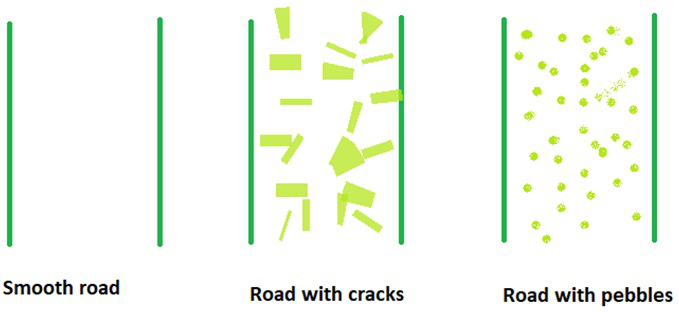### Shape/design of the object

The shape and design of the object determine the surface area in contact with the surface on which it is moving. For example- if the shape of the body is streamlined so while traveling in water or air it will experience a lesser frictional force opposing its motion than any other shape.

It is the area of contact which matters, not the overall area of the surface something is moving on. If a ball of diameter 8 cm is rolling in a small room or a stadium, it makes no difference as long as the floor material and the speed of rolling is the same. Also, if a body has the shortest point of contact then the frictional force generated is also very less as compared to sliding the object it is preferred to roll the object for lesser friction.

So if two objects are in motion (considering they are made of the same material and shape are moving at the same speed) then the larger body will suffer greater friction due to its larger area of contact which will generate a relatively higher frictional force or opposing force relative to its motion.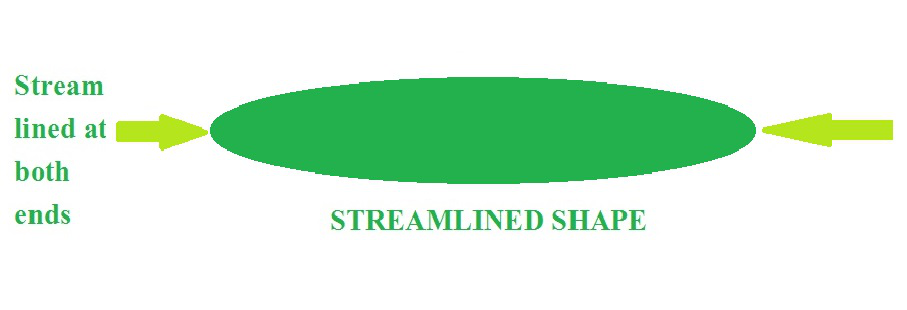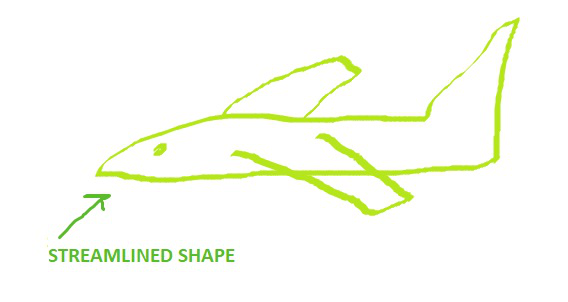### Normal force acting upon the sliding bodies

Force of friction or the opposing force restricting the motion of the body also depends on the Normal Force acting upon the sliding bodies. We usually think of the normal force as opposite to weight by following the Newtonian action-reaction pair. So higher the mass or weight of the object higher will the reaction or normal force generated. As the strength and magnitude of the normal force generated perpendicularly increases which is the force between the “sliding” surfaces, the strength or magnitude of the frictional force or opposing force also increases.

Frictional force or opposing force is a force that opposes two objects sliding against each other, and it acts as a contact force like the normal force. While the normal force acts perpendicular to the flat surface, friction acts in a direction which is along the flat surface of an object.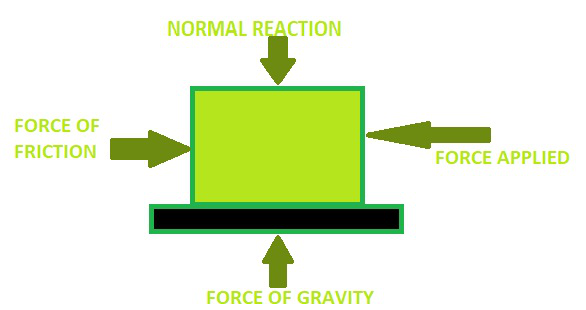### Coefficient of friction

The force of friction depends upon the coefficient of friction of the surface. The greater is the coefficient of friction of the surface the more is the force required to move it over that surface. The coefficient of friction, µ, is the amount of friction existing between two surfaces. A low value of the coefficient of friction indicates that the force required to slide the object is less than the force required when the coefficient of friction is high. The force of friction has the following formula:

F = µ N

Where F= force of friction

µ = Coefficient of friction

N = normal reaction force

### Sample problems

Question 1: What is friction?

Friction, force that resists the sliding or rolling of one solid object over another. Frictional forces, such as the traction needed to walk without slipping, may be beneficial, but they also present a great measure of opposition to motion.

Friction produces unnecessary heat leading to the wastage of energy. The force of friction acts in the opposite direction of motion, so friction slows down the motion of moving objects. Forest fires are caused due to the friction between tree branches.

Question 3: What are the causes of friction?

Friction is a resistive force, which comes into play when there is a relative motion between two bodies in contact. The frictional force between two bodies depends mainly on three factors: (I) the adhesion between body surfaces (ii) roughness of the surface (iii) deformation of bodies.

Question 4: What is streamlined shape explain with example?

A special shape of the body that reduces fluid friction is called the streamlined shape. Example: Aquatic animals like fish, crocodiles, and sharks and the vehicles like cars, flights, ships act. Streamlined shapes help reduce the amount of air drag when an object is moving.

Question 5: Does friction depend on the surface area?

Friction is a contact force when two surfaces interact. Hence, so friction depends on the surface of contact as the surface of contact increases the frictional force also increases.

Question 6: Find the force of friction if the coefficient of friction is 0.25 and the normal reaction offered by the surface is 5N.

Solution:

As we know that, F = µ N

Given, µ = 0.25

N = 5N

F = µ N

Therefore, F = 0.25 × 5N

Therefore, F = 1.25N

Hence, the frictional force exerted is 1.25 N.

Question 7: What is the coefficient of friction if the frictional force exerted is 125N and the normal reaction force offered is 200N.

Solution:

As we know that

F = µ N

Given, F = 125N

N= 200Nµ = 0.625

Hence, the coefficient of friction is 0.625.

Question 8: What is the normal reaction offered by the surface if the frictional force generated was 60N and the coefficient of friction to be 0.6.

Solution:

As we know that

F = µ N

Given, F = 60N

µ = 0.6Hence, the normal reaction generated by the surface is 100N.

My Personal Notes arrow_drop_up